# Simplifying Fractions

Page 2 of 3
Let's try one:

Let's reduce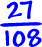What goes into the numerator AND the denominator?

3 goes in... So, let's divide it out!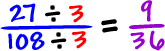Hey, now it's easy! Do you see that 9 goes in?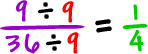so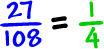The best way to do it is to get the GCF (greatest common factor) of 27 and 108...

 The factors of 27: 1, 3, 9, 27 The factors of 108: 1, 2, 3, 4, 6, 9, 12, 18, 27, 36, 54, 108

The GCF is 27.

If we divide by the GCF, we can do it in one shot: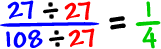But, you know what?

If you can't find the GCF, just start dividing out stuff you see like we did at first.

Head on over to the next page to try some fraction practice problems...